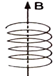A particle having a charge of  100μC and a mass of 10 mg is projected in a uniform magnetic field of 25 mT with a speed of 10 ms−1.  If the velocity is perpendicular to the magnetic field, how long will it take for the particle to come back to its original position for the first time after being projected.

# A particle having a charge of  $100\mathrm{\mu C}$ and a mass of 10 mg is projected in a uniform magnetic field of 25 mT with a speed of   If the velocity is perpendicular to the magnetic field, how long will it take for the particle to come back to its original position for the first time after being projected.

1. A

27s

2. B

28s

3. C

25s

4. D

26s

Register to Get Free Mock Test and Study Material

+91

Verify OTP Code (required)

### Solution:

The particle moves along a circle and returns to its original position after completing the circle that is after one time period. The time period is $\mathrm{T}=\frac{2\mathrm{\pi m}}{\mathrm{qB}}$
$=\frac{2\pi ×\left(10×{10}^{-6}\mathrm{kg}\right)}{\left(100×{10}^{-6}\mathrm{C}\right)×\left(25×{10}^{-3}\mathrm{T}\right)}=25\mathrm{s}$
If the velocity of the charge is not perpendicular to the magnetic field, we can break the velocity in two components-${\mathrm{v}}_{\parallel }$ , parallel to the field and ${\mathrm{v}}_{\perp },$  perpendicular to the field. The component ${\mathrm{v}}_{\parallel }$  remains unchanged as the force $\mathrm{q}\stackrel{\to }{\mathrm{v}}×\stackrel{\to }{\mathrm{B}}$  is perpendicular to it. In the plane perpendicular to the field, the particle traces a circle of radius $\mathrm{r}=\frac{{\mathrm{mv}}_{\perp }}{\mathrm{qB}}$ as given by equation. The resultant path is a helixRegister to Get Free Mock Test and Study Material

+91

Verify OTP Code (required)